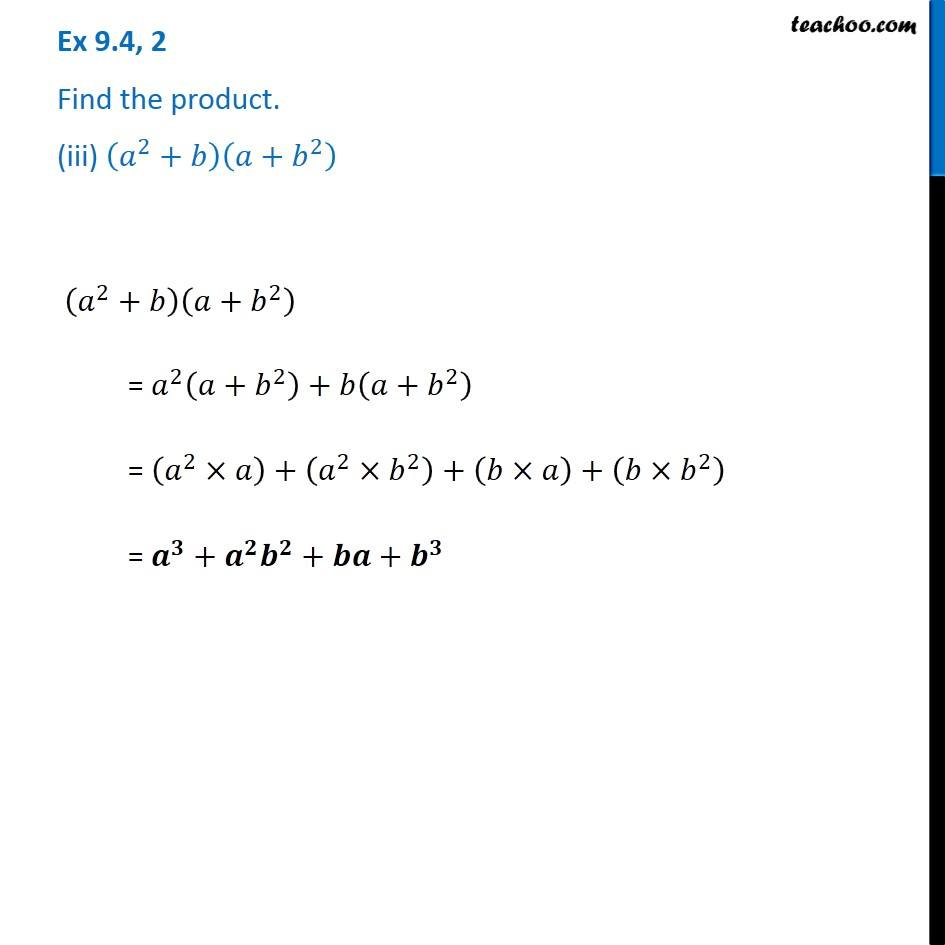Ex 9.4

Chapter 9 Class 8 Algebraic Expressions and Identities
Serial order wiseGet live Maths 1-on-1 Classs - Class 6 to 12

### Transcript

Ex 9.4, 2 Find the product. (iii) (𝑎^2+𝑏)(𝑎+𝑏^2 ) (𝑎^2+𝑏)(𝑎+𝑏^2 ) = 𝑎^2 (𝑎+𝑏^2 )+𝑏(𝑎+𝑏^2 ) = (𝑎^2×𝑎)+(𝑎^2×𝑏^2 )+(𝑏×𝑎)+(𝑏×𝑏^2 ) = 𝒂^𝟑+𝒂^𝟐 𝒃^𝟐+𝒃𝒂+𝒃^𝟑